Note: This is an archvied version of our old webpage. Some links might be broken. The current one can be found here.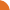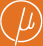Chair for Foundations of Software Reliability and Theoretical Computer Science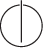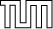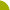Publications - On the Complexity of the Equivalence Problem for Probabilistic AutomataReference:

Stefan Kiefer, Andrzej S. Murawski, Joël Ouaknine, Björn Wachter, and James Worrell. On the complexity of the equivalence problem for probabilistic automata. Technical report, arXiv.org, January 2012. Available at http://arxiv.org/abs/1112.4644.

Abstract:

Deciding equivalence of probabilistic automata is a key problem for establishing various behavioural and anonymity properties of probabilistic systems. In recent experiments a randomised equivalence test based on polynomial identity testing outperformed deterministic algorithms. In this paper we show that polynomial identity testing yields efficient algorithms for various generalisations of the equivalence problem. First, we provide a randomized NC procedure that also outputs a counterexample trace in case of inequivalence. Second, we consider equivalence of probabilistic cost automata. In these automata transitions are labelled with integer costs and each word is associated with a distribution on costs, corresponding to the cumulative costs of the accepting runs on that word. Two automata are equivalent if they induce the same cost distributions on each input word. We show that equivalence can be checked in randomised polynomial time. Finally we show that the equivalence problem for probabilistic visibly pushdown automata is logspace equivalent to the problem of whether a polynomial represented by an arithmetic circuit is identically zero.

Suggested BibTeX entry:

@techreport{12KMOWW:FOSSACS-TR,
author = {Stefan Kiefer and Andrzej S. Murawski and Jo\"el Ouaknine and Bj\"orn Wachter and James Worrell},
institution = {arXiv.org},
month = {January},
note = {Available at http://arxiv.org/abs/1112.4644},
title = {On the Complexity of the Equivalence Problem for Probabilistic Automata},
year = {2012}
}PDF (206 kB)See arxiv.org ...Conference version